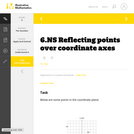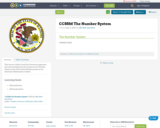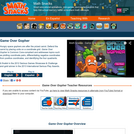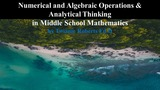Updating search results...

# 10 Results

View
Selected filters:
• MCCRS.Math.Content.6.NS.C.6bUnrestricted Use
CC BY
Rating
0.0 stars

This is a task from the Illustrative Mathematics website that is one part of a complete illustration of the standard to which it is aligned. Each task has at least one solution and some commentary that addresses important aspects of the task and its potential use.

Subject:
Mathematics
Numbers and Operations
Material Type:
Activity/Lab
Provider:
Illustrative Mathematics
Provider Set:
Illustrative Mathematics
Author:
Illustrative Mathematics
08/06/2015Conditional Remix & Share Permitted
CC BY-SA
Rating
0.0 stars

This resource links to both the Fractions progression document published by the Common Core Writing Teams in June 2011 and the Module posted on the Illustrative Mathematics website.

Material Type:
Assessment
Homework/Assignment
Teaching/Learning Strategy
08/08/2019Some Rights Reserved
Rating
0.0 stars

Game Over Gopher is an exciting tower defense game that guides students in plotting coordinate pairs, differentiating negative coordinates from positive coordinates, and identifying the four quadrants. Hungry space gophers are marching towards a prize carrot, and to defend it players place tools around the coordinate grid to “feed” gophers and make them lose interest. Ruby mines (which must be placed at designated x, y coordinates) yield currency that players spend to strategically place carrot launchers, garlic rays, corn silos, and beet traps – gopher feeding tools. To introduce students to the coordinate grid, Game Over Gopher introduces new skills via interactive tutorials and uses consistent visual clues (e.g., x red, y blue) to guide players in plotting coordinates. This means it’s not necessary to teach students coordinate plotting ahead of time. As the levels progress, the number of tools available increases, the level of math vocabulary increases, the scale of the grid changes, and players are asked to expand their mastery of the grid by reflecting points across axes. The game lowers intimidation about the coordinate grid, helps students understand how positive and negative numbers reflect each other across the axes, and helps students get comfortable with the four quadrants.

Subject:
Mathematics
Material Type:
Game
Interactive
Provider:
Learning Games Lab
Author:
NMSU Learning Games Lab
07/20/2015Conditional Remix & Share Permitted
CC BY-NC
Rating
0.0 stars
Rating
0.0 stars
Subject:
Mathematics
Material Type:
Full Course
Provider:
Pearson
10/06/2016Conditional Remix & Share Permitted
CC BY-NC
Rating
0.0 stars

Rational Numbers

Type of Unit: Concept

Prior Knowledge

Students should be able to:

Solve problems with positive rational numbers.
Plot positive rational numbers on a number line.
Understand the equal sign.
Use the greater than and less than symbols with positive numbers (not variables) and understand their relative positions on a number line.
Recognize the first quadrant of the coordinate plane.

Lesson Flow

The first part of this unit builds on the prerequisite skills needed to develop the concept of negative numbers, the opposites of numbers, and absolute value. The unit starts with a real-world application that uses negative numbers so that students understand the need for them. The unit then introduces the idea of the opposite of a number and its absolute value and compares the difference in the definitions. The number line and positions of numbers on the number line is at the heart of the unit, including comparing positions with less than or greater than symbols.

The second part of the unit deals with the coordinate plane and extends student knowledge to all four quadrants. Students graph geometric figures on the coordinate plane and do initial calculations of distances that are a straight line. Students conclude the unit by investigating the reflections of figures across the x- and y-axes on the coordinate plane.

Subject:
Mathematics
Numbers and Operations
Material Type:
Unit of Study
Provider:
PearsonConditional Remix & Share Permitted
CC BY-NC
Rating
0.0 stars

Students play a game in which they try to find dinosaur bones in an archaeological dig simulator. The players guess where the bones are on the coordinate plane using hints and reasoning.Key ConceptsThe coordinate plane consists of a horizontal number line and a vertical number line that intersect at right angles. The point of intersection is the origin, or (0,0). The horizontal number line is often called the x-axis. The vertical number line is often called the y-axis.A point&rsquo;s location on the coordinate plane can be described using words or numbers. Ordered pairs name locations on the coordinate plane. To find the location of the ordered pair (m,n), first locate m on the x-axis and draw a vertical line through this point. Then locate n on the y-axis and draw a horizontal line through this point. The intersection of these lines is the location of (m,n).The coordinate plane is divided into four quadrants:Quadrant I: (+,+)Quadrant II: (&minus;,+)Quadrant III: (&minus;,&minus;)Quadrant IV: (+,&minus;)Goals and Learning ObjectivesName locations on the coordinate plane.

Subject:
Numbers and Operations
Material Type:
Lesson Plan
09/21/2015Conditional Remix & Share Permitted
CC BY-NC
Rating
0.0 stars

Students draw a figure on the coordinate plane that matches a written description.Key ConceptsOrdered pairs name locations on the coordinate plane. The first coordinate tells how many units to go left or right of the origin (0,0) along the x-axis. The second coordinate tells how many units to go up or down from the origin along the y-axis.Goals and Learning ObjectivesDraw a figure that matches a description of a figure on the coordinate plane.Give coordinates of points on the coordinate plane.Write descriptions of figures on the coordinate plane.

Subject:
Numbers and Operations
Material Type:
Lesson Plan
09/21/2015Conditional Remix & Share Permitted
CC BY-NC
Rating
0.0 stars

Students reflect a figure across one of the axes on the coordinate plane and name the vertices of the reflection. As they are working, students look for and make use of structure to identify a convention for naming the coordinates of the reflected figure.Key ConceptsWhen point (m,n) is reflected across the y-axis, the reflected point is (&minus;m,n).When point (m,n) is reflected across the x-axis, the reflected point is&nbsp;(m,&minus;n).When point (m,n) is reflected across the origin (0,0), the reflected point is (&minus;m,&minus;n).Goals and Learning ObjectivesReflect a figure across one of the axes on the coordinate plane.Name the vertices of the reflected figure.Discern a pattern in the coordinates of the reflected figure.

Subject:
Numbers and Operations
Material Type:
Lesson Plan
09/21/2015Educational Use
Rating
0.0 stars

The purpose of this lesson is to teach students about the three dimensional Cartesian coordinate system. It is important for structural engineers to be confident graphing in 3D in order to be able to describe locations in space to fellow engineers.

Subject:
Applied Science
Astronomy
Engineering
Physical Science
Material Type:
Activity/Lab
Lesson Plan
Provider:
TeachEngineering
Provider Set:
TeachEngineering
Author:
Ben Burnham
09/18/2014Unrestricted Use
CC BY
Rating
0.0 stars

This supplemental resource provides problems and activities related to&nbsp;Numerical and Algebraic Operations &amp; Analytical Thinking in Middle School Mathematics.

Subject:
Mathematics
Material Type:
Activity/Lab
Author:
Twianie Roberts Ed.D